Toda Lattice Solitons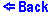Toda lattice is a monatomic chain of particles of mass m with the following interaction between nearest-neighbors
V(r) = (a/b)exp(-br)+ar-a/b ,
where r is the deviation of the nearest-neighbor distance from its equilibrium value, and ab > 0.

Such lattice supports soliton solutions. For a single soliton the deviation of the nth particle from its equilibrium position can be written as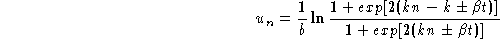,
where the lattice constant is chosen as the unit of length, and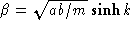.

The soliton is a pulslike wave with the speed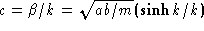. It always moves faster than plane waves. (The speed of sound is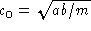.) The smaller the soliton's width (~1/k), the faster it propagates.

The lattice is compressed by the value 2k/b around a soliton (we assume b > 0). Due to such a compression, we can speak of the soliton's "mass" = 2mk/b.

A more complete description of the Toda solitons you can find in the book:
M. Toda, Theory of Nonlinear Lattices, (Springer-Verlag, New York 1989).

The above applet allows you to watch propagating solitons in the lattice of 15 particles with fixed boundaries. The evolution of the chain is calculated by the molecular-dynamics technique. The parameters of the lattice are the following: a=1, b=1, m=1.

You can launch either a wide soliton (k=0.5) or a sharp one (k=1). You can also see non-destructive collisions of two sharp solitons.

The time is shown in units of the shortest period of small amplitude plane wave vibrations,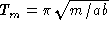. Energy is shown in arbitrary units. The kinetic energy of the particle and the potential energy of the bond are shown as the red and the yellow bars, respectively.

If you wait for a while you will see a power spectrum of the particles' vibrations. It will be shown in the left panel. As the time of the evolution goes the spectrum resolution improves. The frequency unit is the maximal plane wave frequency,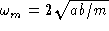.

Last modified: December 1, 1996
Sergey Kiselev, kiselev@msc.cornell.edu